00:00:00

# What is this course about?

• Learn Python syntax
• Learn control structure such as `if .. else` and `for`
• Learn basic data structures such as `list` and `dict`
• Learn how to define and use functions in Python
• Must have completed the pre-requisite course `Bootstrapping Python`
• Will use the `turtle` library in Jupyter notebook

# Importing a library

Python offers a rich, vast collection of libraries to its users. Some of these libraries are built-in, while others require installation. To use a library, we first need to import it.

 ```1 2 3 4``` ```from turtle import Turtle, setup, clearscreen setup(width=500, height=500) clearscreen() tom = Turtle() ```

Sometimes you see people import this way:

 ```1 2 3 4``` ```import turtle turtle.setup(width=500, height=500) turtle.clearscreen() tom = turtle.Turtle() ```
• In Jupyter notebook, once you imported a library in one cell, the library becomes available to subsequent cell evaluations.

# Tom the Turtle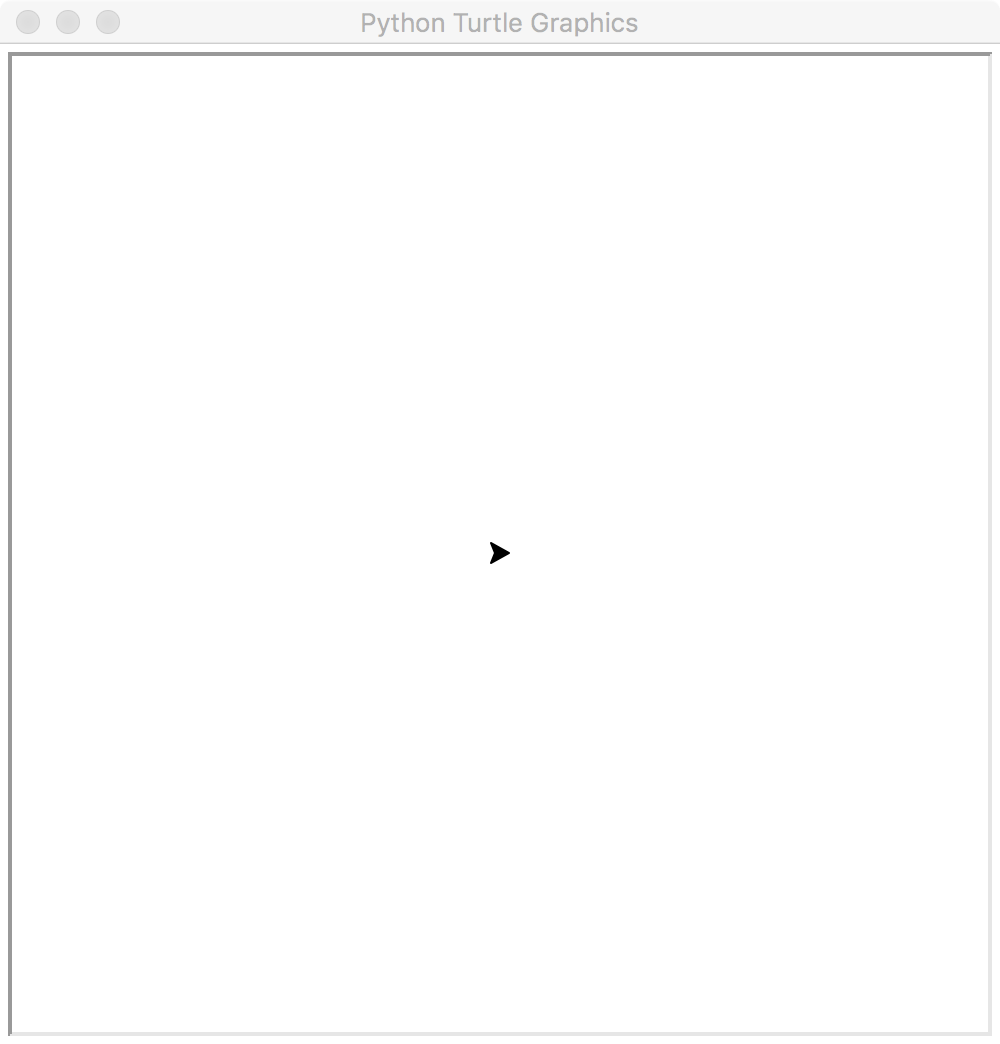# Declaring a variable and assigning a value to it

A variable in Python is similar to one in algebra - it holds a value, and can hold any value.

 `1` ```tom = Turtle() ```
• `tom` is a variable, `=` the assignment operator, and `Turtle()` the value assigned.
• The Python interpreter understands `Turtle` only because we imported `Turtle` in the previous line.
• The value `Turtle()` is an object of the `Turtle` class. An object packs a lot of functionalities inside it.

# Manipulating an object

You make an object do things by calling its methods (or functions).

 ```1 2 3 4 5``` ```tom = Turtle() tom.forward(100) tom.right(90) tom.forward(100) tom.right(90) ```
• `forward` and `right` are methods (or functions) of the `Turtle` class.
• Methods can have parameters. The `forward` method's parameter specifies the distance to go. The `right` method's parameter specifies the degrees of angle to turn.

# The Turtle moves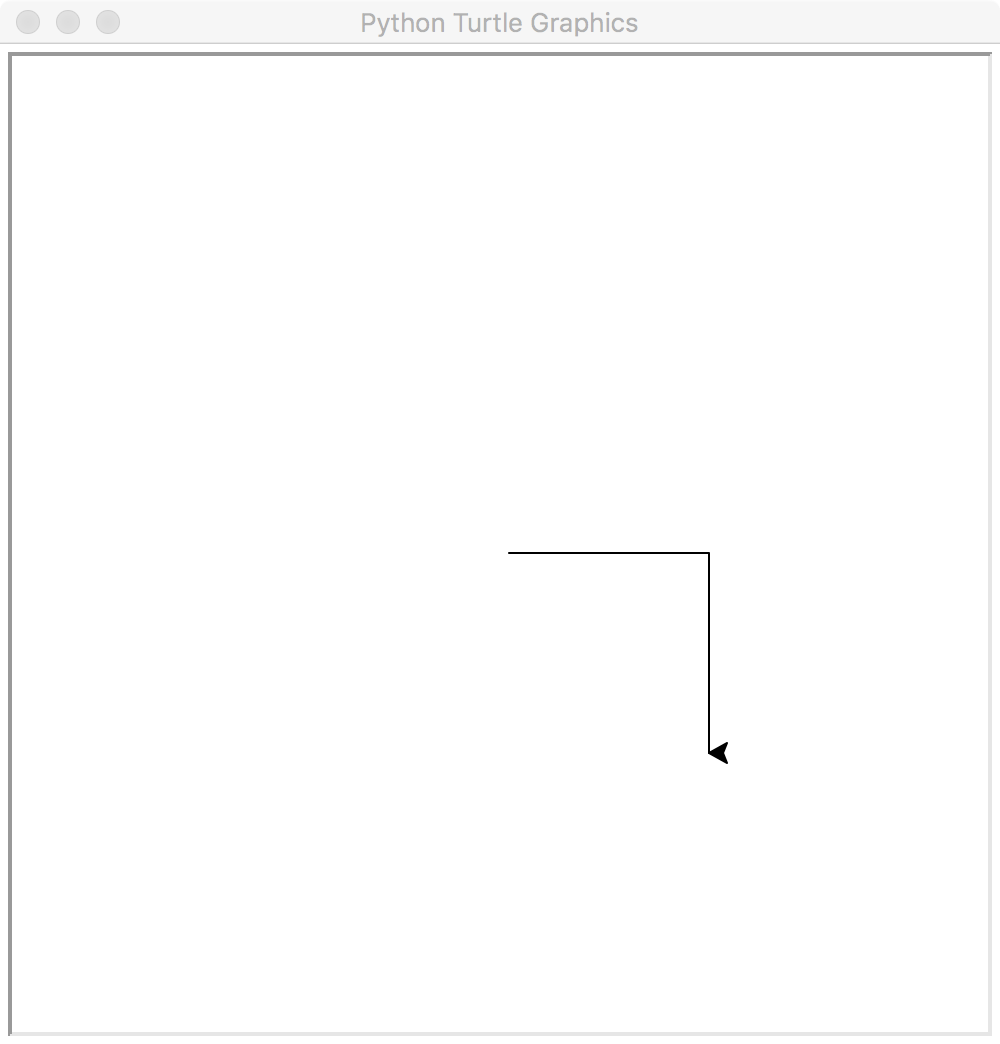# Speeding up the Turtle

 ```1 2 3 4 5 6 7 8``` ```tom = Turtle() # Sets the speed (0-10) of the turtle # 1: slow, 5: normal, 10: fast, 0: fastest tom.speed(0) tom.forward(100) tom.right(90) tom.forward(100) tom.right(90) ```
• Any text following a '#' character is ignored by the Python interpreter. People use '#' to comment their Python code.
• It's a good practice to comment your code, because it helps others (and future you) understand your code better.

# Exercise: Can you draw a square?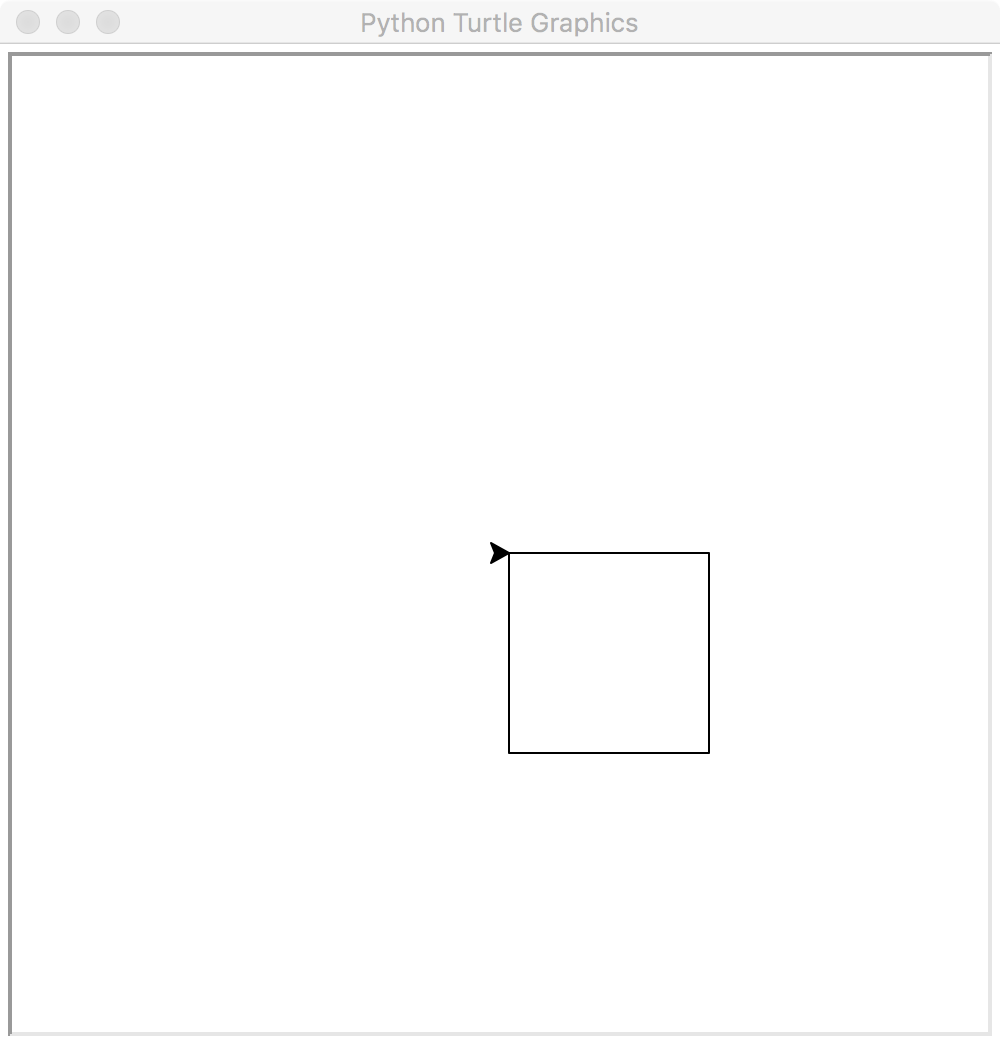# Answer:

Note: this is just one of the many possible answers

 ``` 1 2 3 4 5 6 7 8 9 10 11 12 13 14 15 16 17 18 19``` ```tom = Turtle() # Sets the speed (0-10) of the turtle tom.speed(0) # the bottom side tom.forward(100) tom.right(90) # the right side tom.forward(100) tom.right(90) # the top side tom.forward(100) tom.right(90) # the left side tom.forward(100) tom.right(90) ```

# Using `for` loop

We can simplify our square drawing with `for` loop.

 ```1 2 3``` ```for i in (1, 2, 3, 4): tom.forward(100) tom.right(90) ```
• Loop helps you repeat a task easily.
• The `i` is called the loop variable - its value is updated at the beginning of each iteration.
• Did you notice the indentation on line 2 and 3? Indentation tells the Python interpreter exactly which lines are included in the loop.

# Loop variable

Try adding the following line in our square-drawing loop

 ```1 2 3 4``` ```for i in (1, 2, 3, 4): tom.write(i) # <-- add this line tom.forward(100) tom.right(90) ```

# The result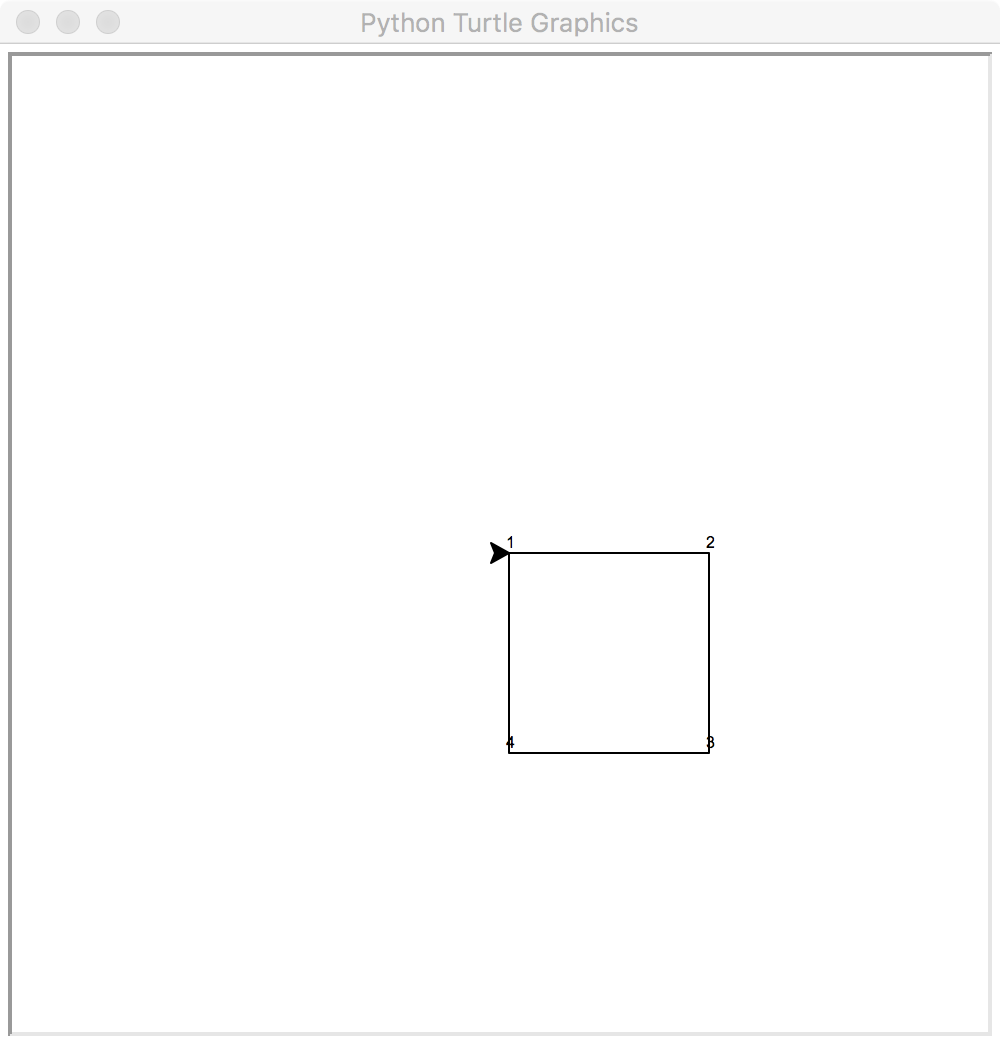# Exercise: Draw a regular octagon

Hint: use 80 as side length so Tom doesn't fall over the canvas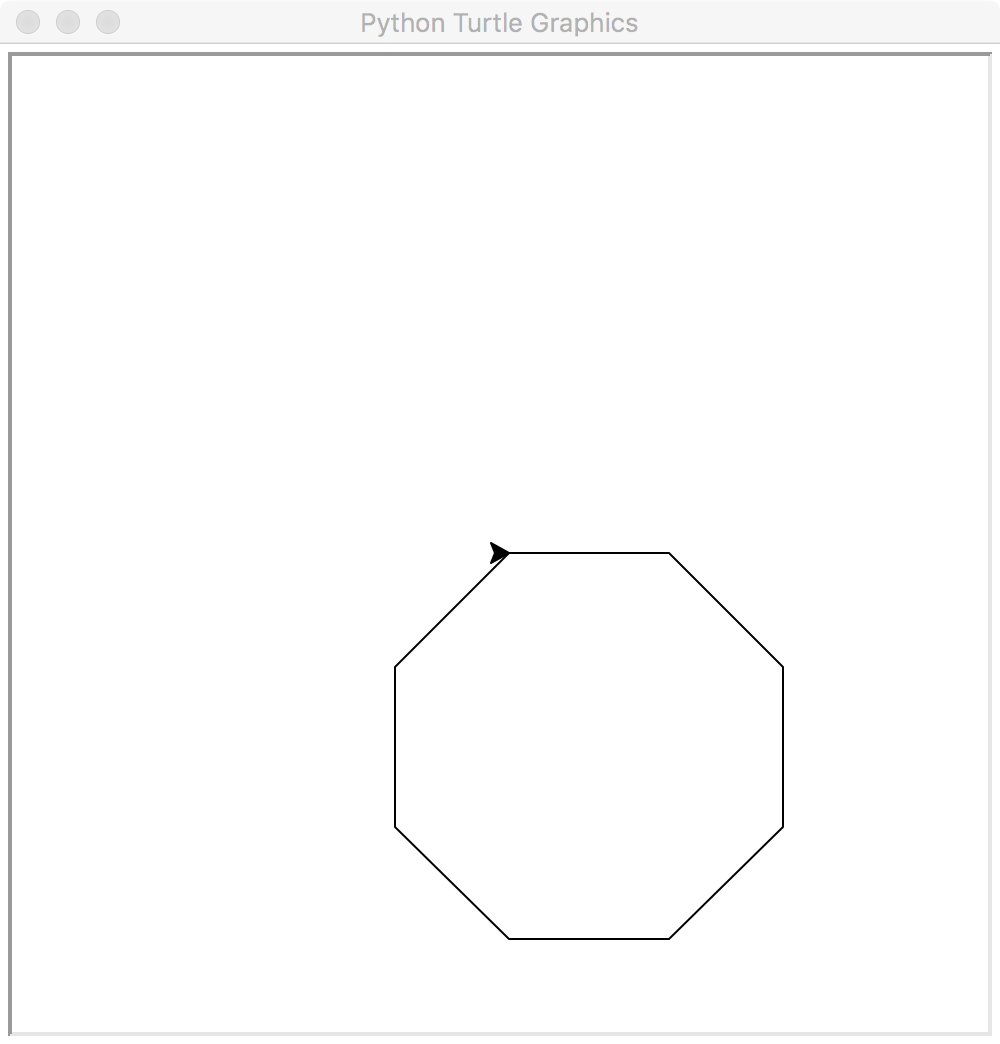# Answer:

 ```1 2 3``` ```for i in (1, 2, 3, 4, 5, 6, 7, 8): tom.forward(80) tom.right(360/8) ```

We can simplify the answer even further by using `range()`

 ```1 2 3``` ```for i in range(1, 9): tom.forward(80) tom.right(360/8) ```

# Exercise: Draw a circle!

Hint: Can you draw a hexadecagon (16-gon) first? What does the result tell you?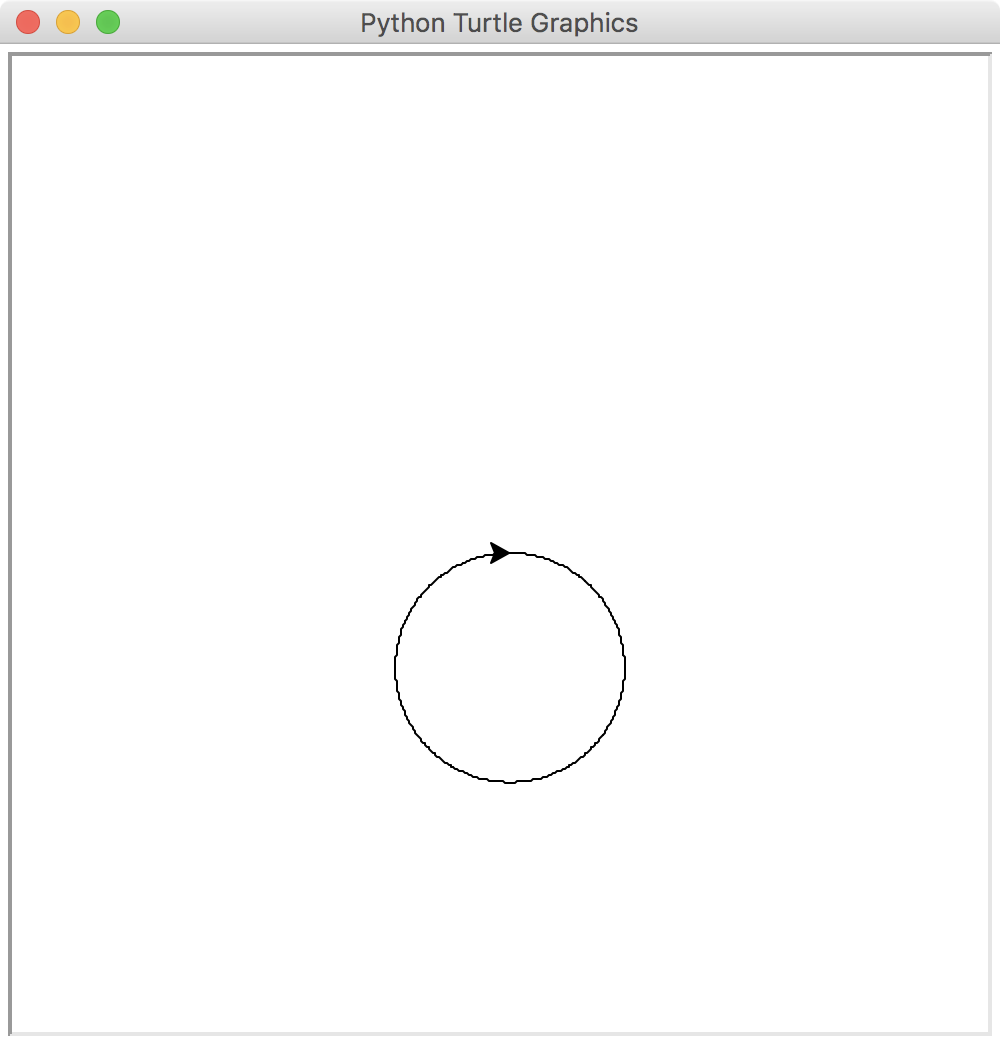# Answer:

 ```1 2 3``` ```for i in range(1, 361): tom.forward(1) tom.right(1) ```

# With flying colors - 1

 ```1 2 3 4``` ```tom.color('red') for i in range(1, 361): tom.forward(1) tom.right(1) ```
• Use Turtle object's `.color()` method set the pen color.

# How it looks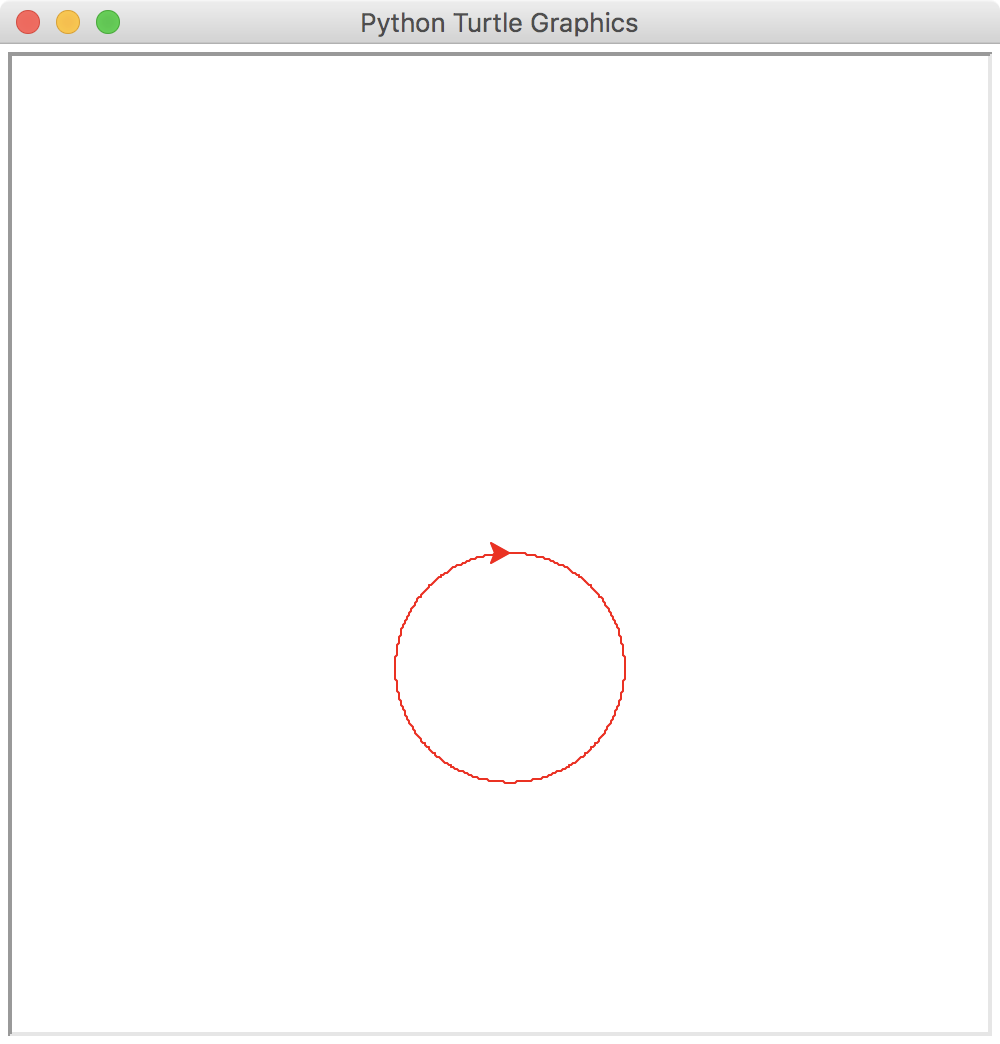# With flying colors - 2!

 ```1 2 3 4 5 6``` ```tom.color('red', 'green') tom.begin_fill() for i in range(1, 361): tom.forward(1) tom.right(1) tom.end_fill() ```
• Turtle object's `.color()` method can also set the fill color.
• Use Turtle object's `.begin_fill()` and `.end_fill()` methods to indicate when filling should begin and end.

# How it looks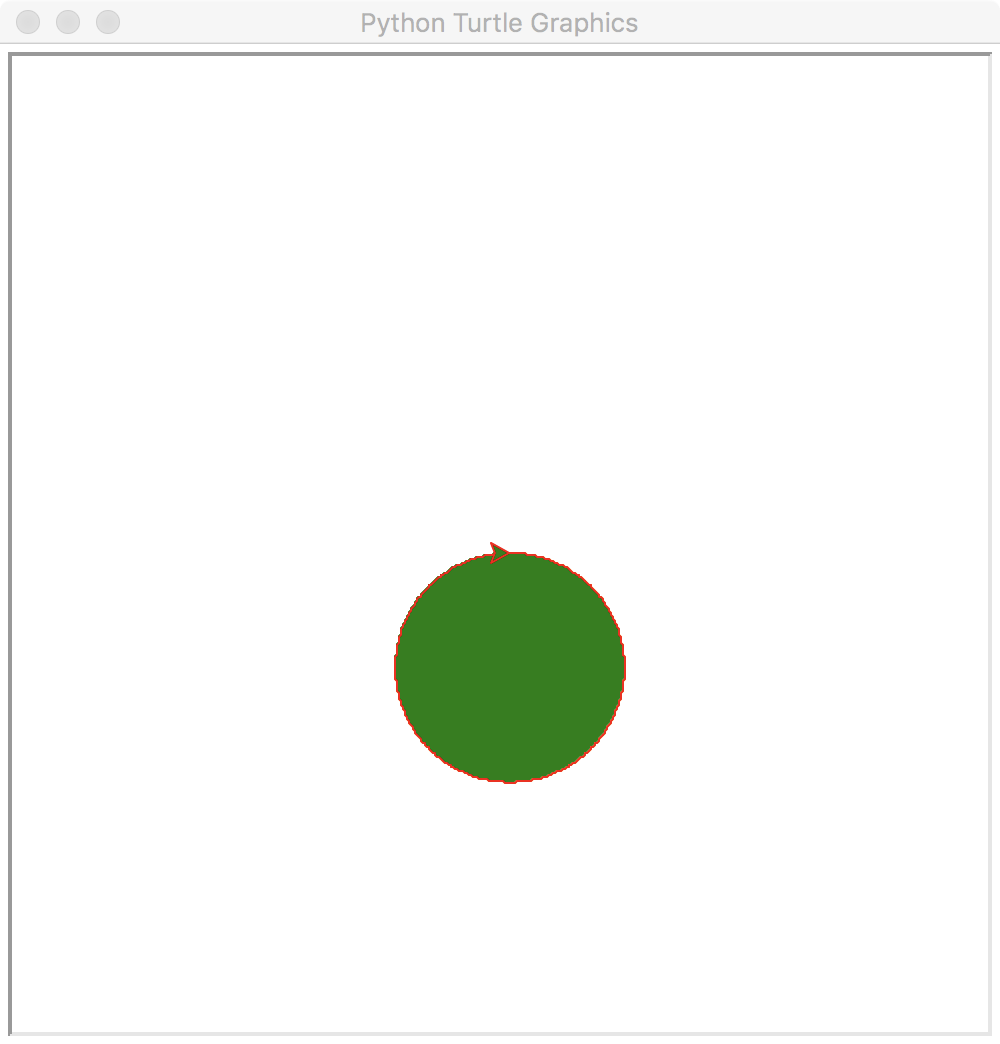# Exercise: Draw a star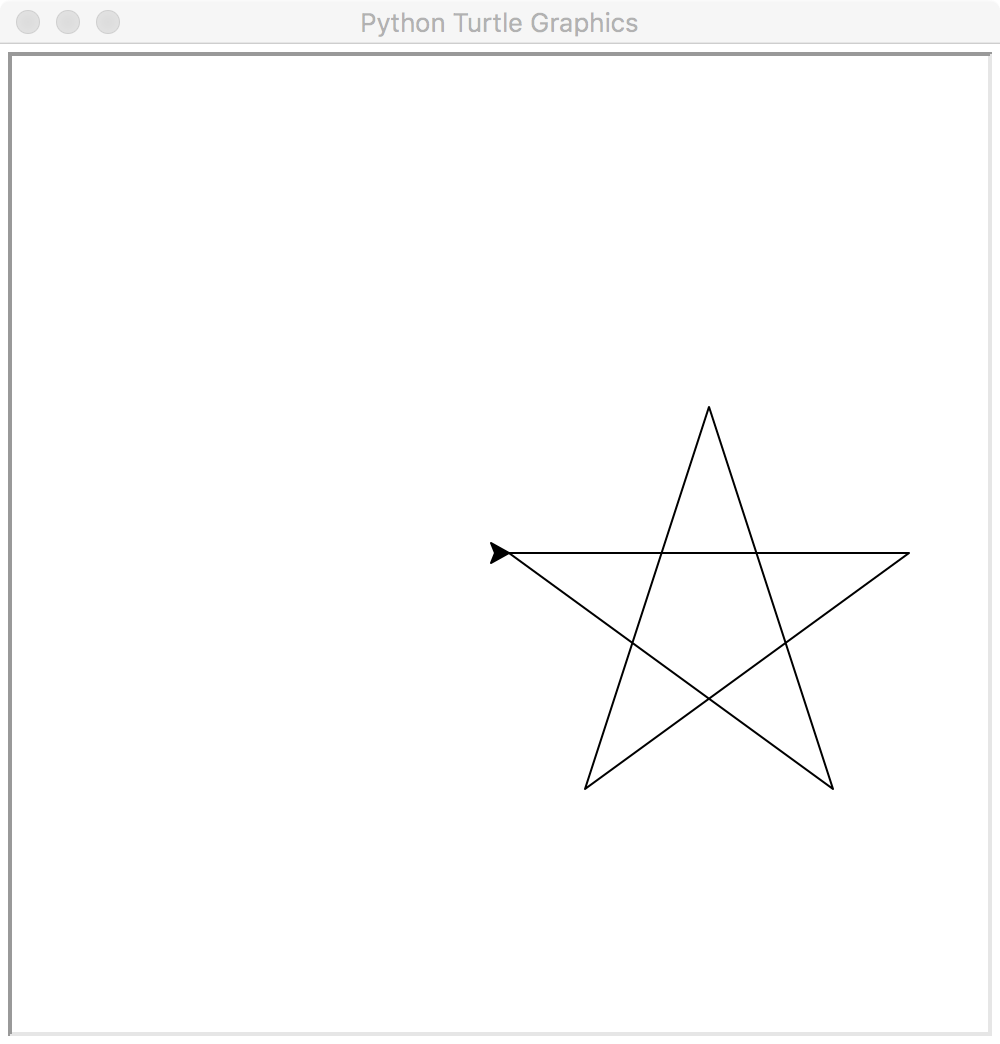# Answer:

 ```1 2 3``` ```for i in range(1, 6): tom.forward(200) tom.right(180-180/5) ```

# Adding colors to our star

 ```1 2 3 4 5 6``` ```tom.color('red', 'blue') tom.begin_fill() for i in range(1, 6): tom.forward(200) tom.right(180-180/5) tom.end_fill() ```

# Houston, we have a problem!

Due to intersecting lines, Turtle is confused about where to shade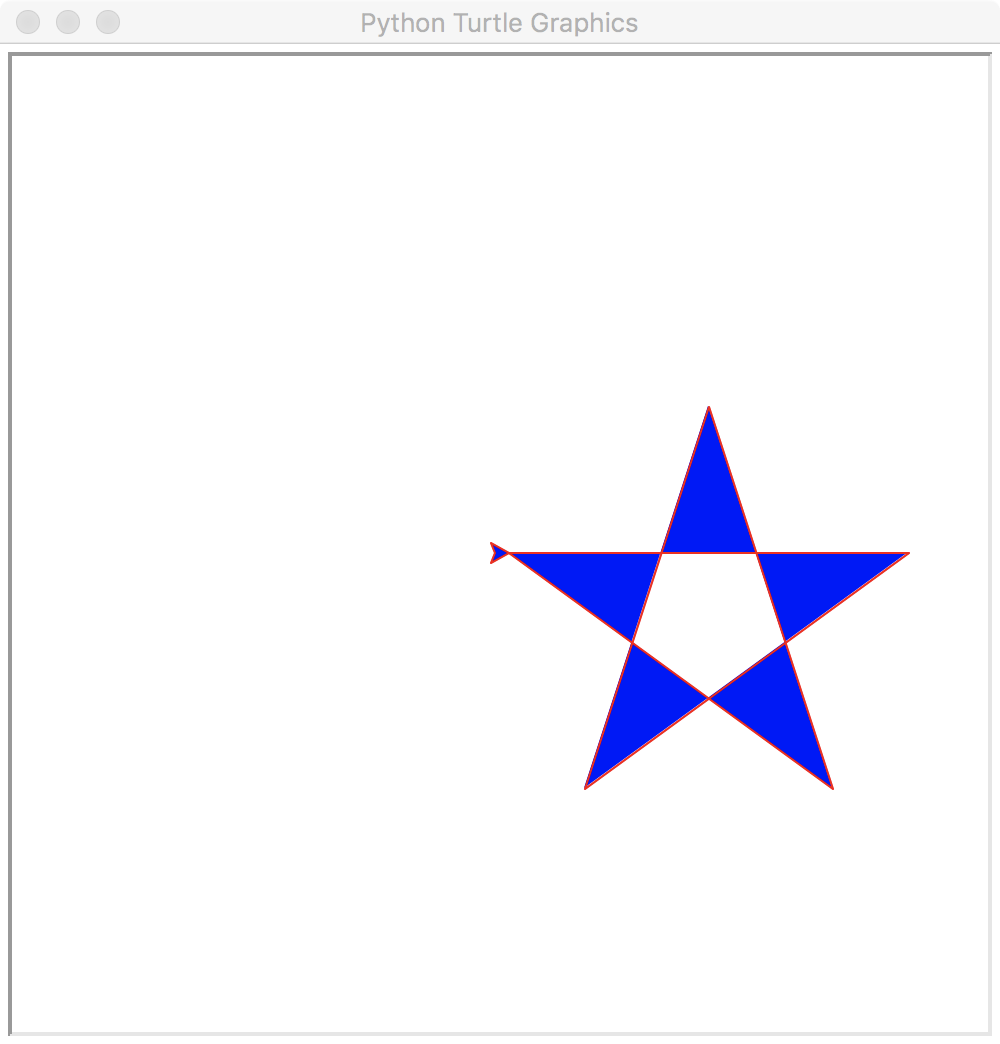# Conditional branching

 ```1 2 3 4 5 6 7 8 9``` ```tom.color('red', 'blue') tom.begin_fill() for i in range(1, 11): tom.forward(80) if i % 2 == 1: tom.left(36*2) else: tom.right(180-36) tom.end_fill() ```
• `if .. else` does one thing or the other, but never both!
• `%` is the modulo operator - it gives the remainder of the left operand divided by the right operand.
• if `i` is a non-negative integer, how many possible values of `i % 2` are there?

# That's solid!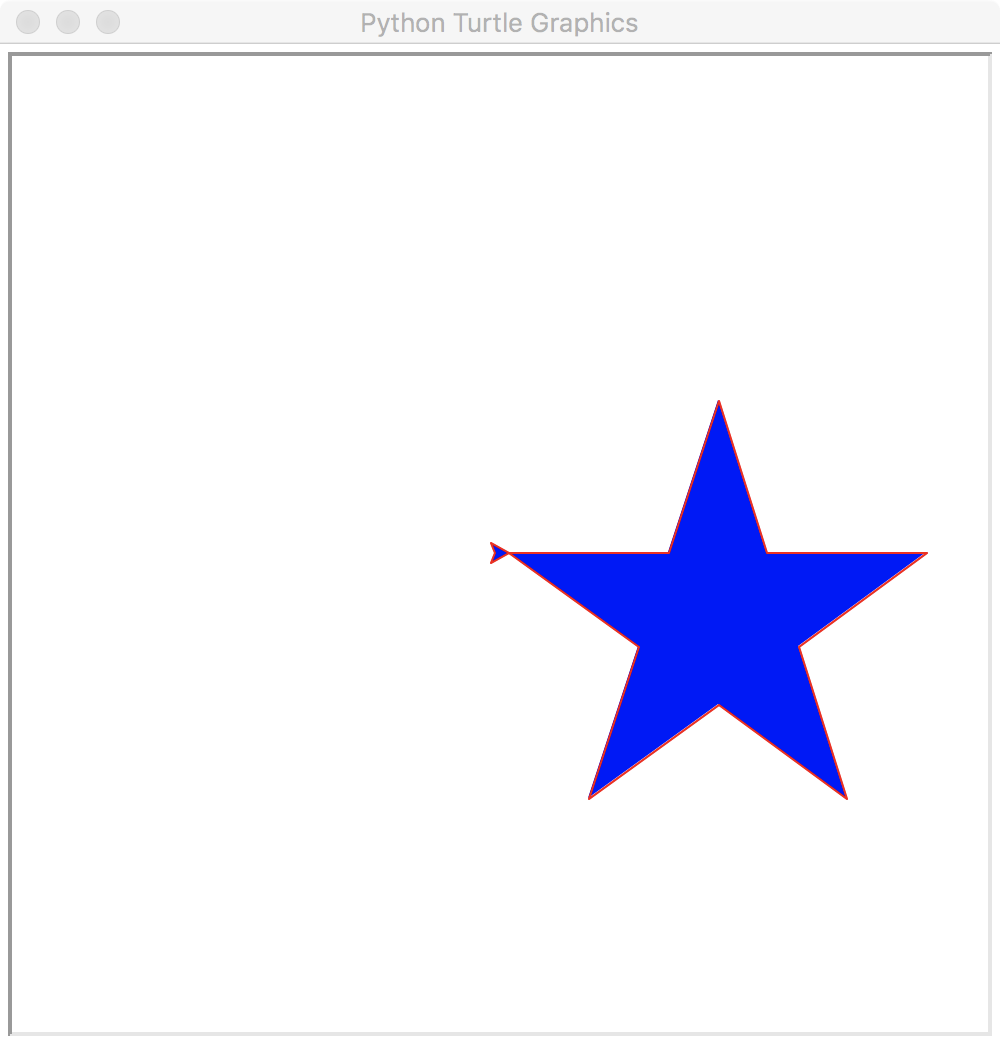# Exercise: Draw a set of stairs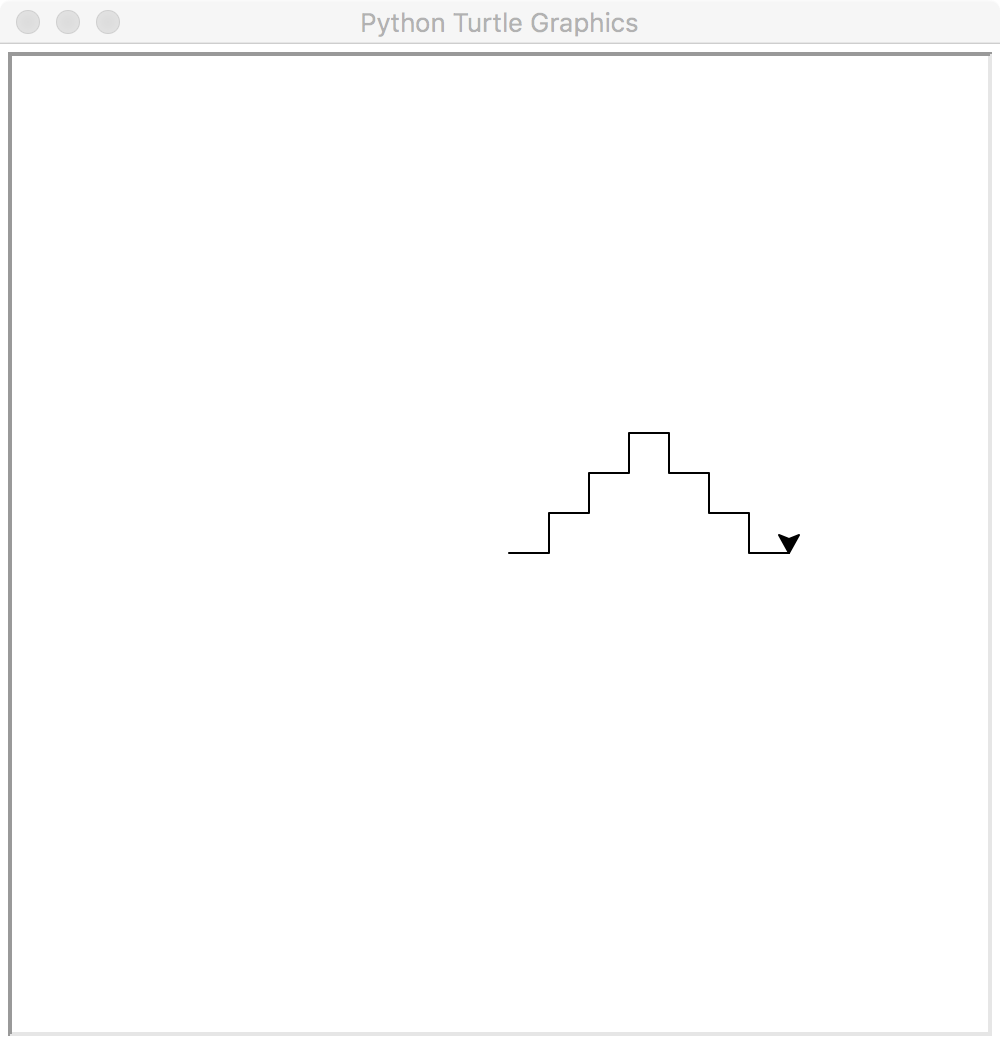# Answer:

 ``` 1 2 3 4 5 6 7 8 9 10 11 12``` ```for i in range(1, 14): tom.forward(20) if i < 7: if i % 2 == 1: tom.left(90) else: tom.right(90) else: if i % 2 == 1: tom.right(90) else: tom.left(90) ```

# Exercise: color top of the stairs red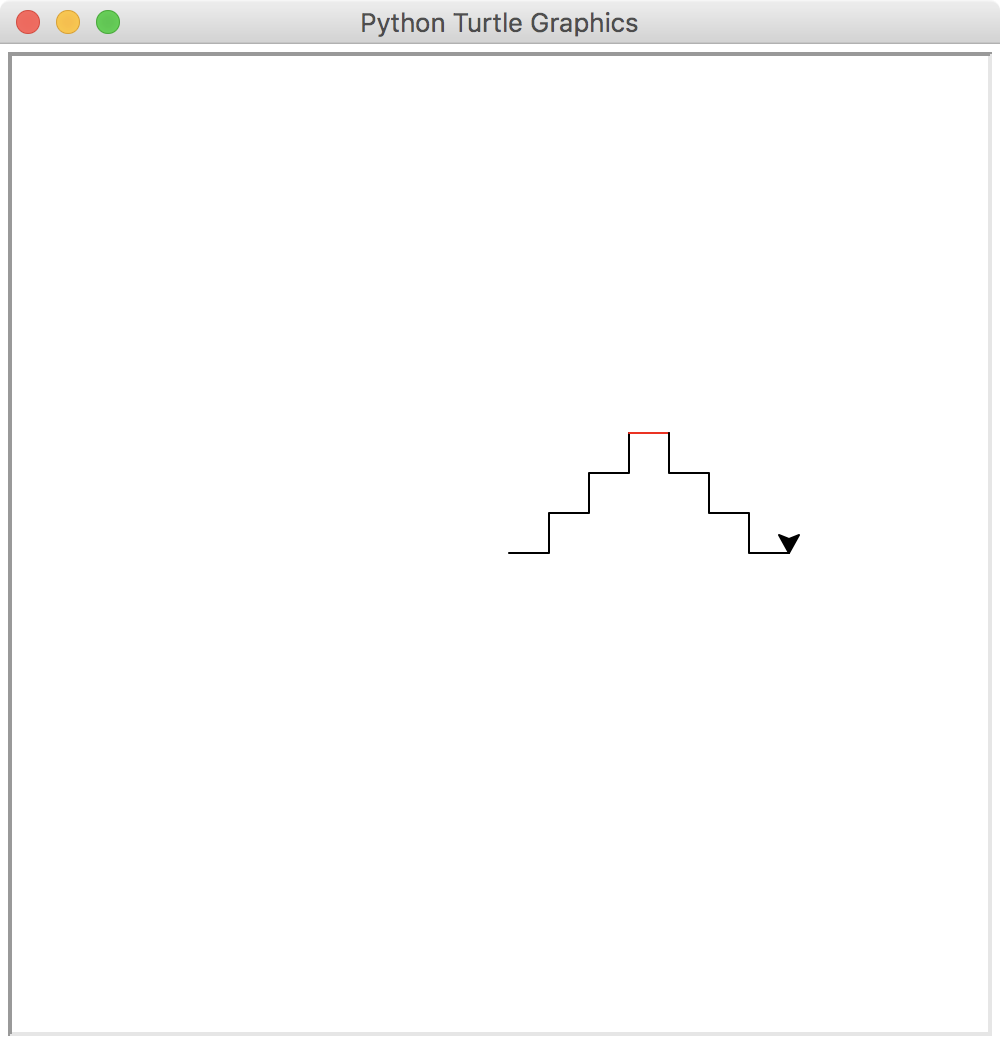# Answer:

 ``` 1 2 3 4 5 6 7 8 9 10 11 12 13 14 15 16``` ```for i in range(1, 14): if i == 7: tom.color('red') else: tom.color('black') tom.forward(20) if i < 7: if i % 2 == 1: tom.left(90) else: tom.right(90) else: if i % 2 == 1: tom.right(90) else: tom.left(90) ```

# Exercise: Draw a house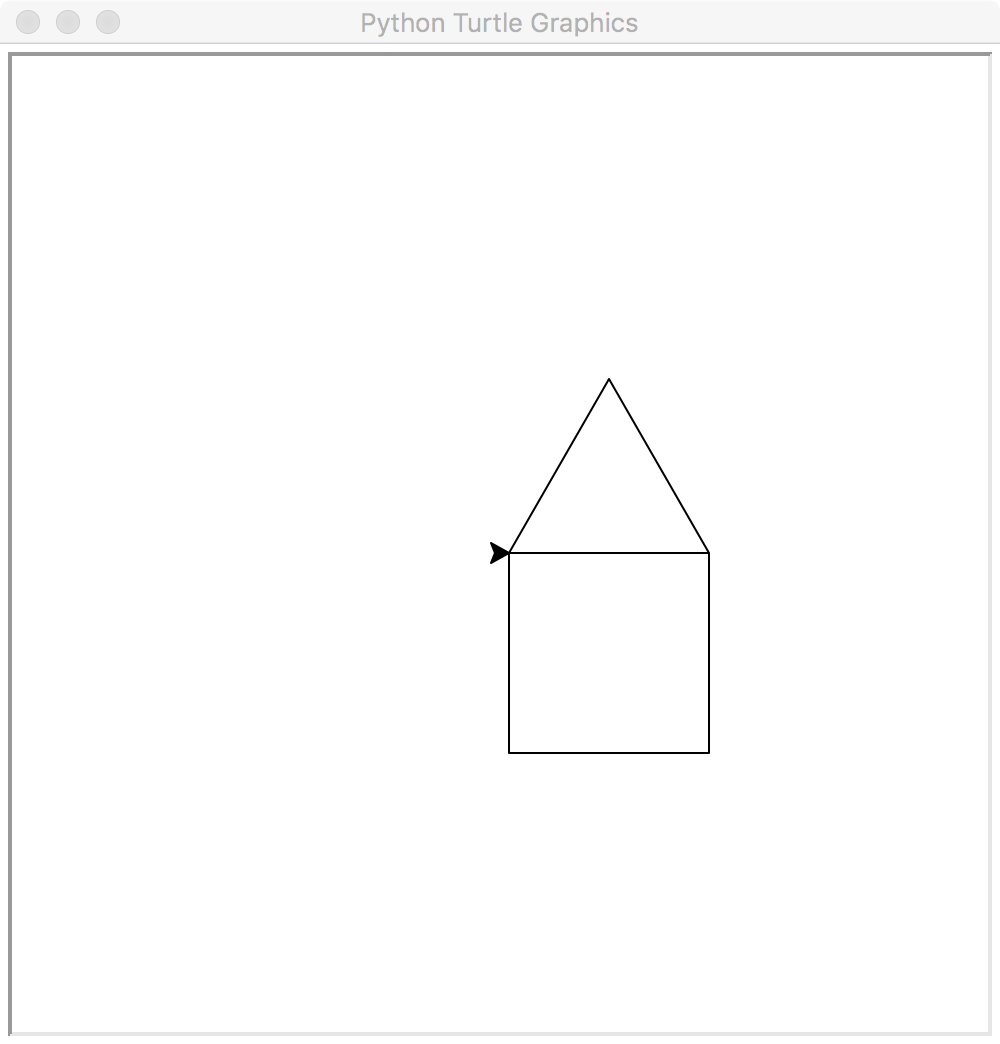# Answer:

 ``` 1 2 3 4 5 6 7 8 9 10 11``` ```tom.fd(100) tom.lt(120) tom.fd(100) tom.lt(120) tom.fd(100) tom.lt(30) tom.fd(100) tom.lt(90) tom.fd(100) tom.lt(90) tom.fd(100) ```

# Introducing functions

How to draw several house with minimum effort? The answer: functions.

 ``` 1 2 3 4 5 6 7 8 9 10 11 12 13 14``` ```def house(): tom.fd(100) tom.lt(120) tom.fd(100) tom.lt(120) tom.fd(100) tom.lt(30) tom.fd(100) tom.lt(90) tom.fd(100) tom.lt(90) tom.fd(100) house() # <-- calling (or invoking) the function ```
• The above code just `def`ined a function called `house`.
• The `house` function has no parameters, as indicated by the empty `()`.

# Exercise: draw a townhouse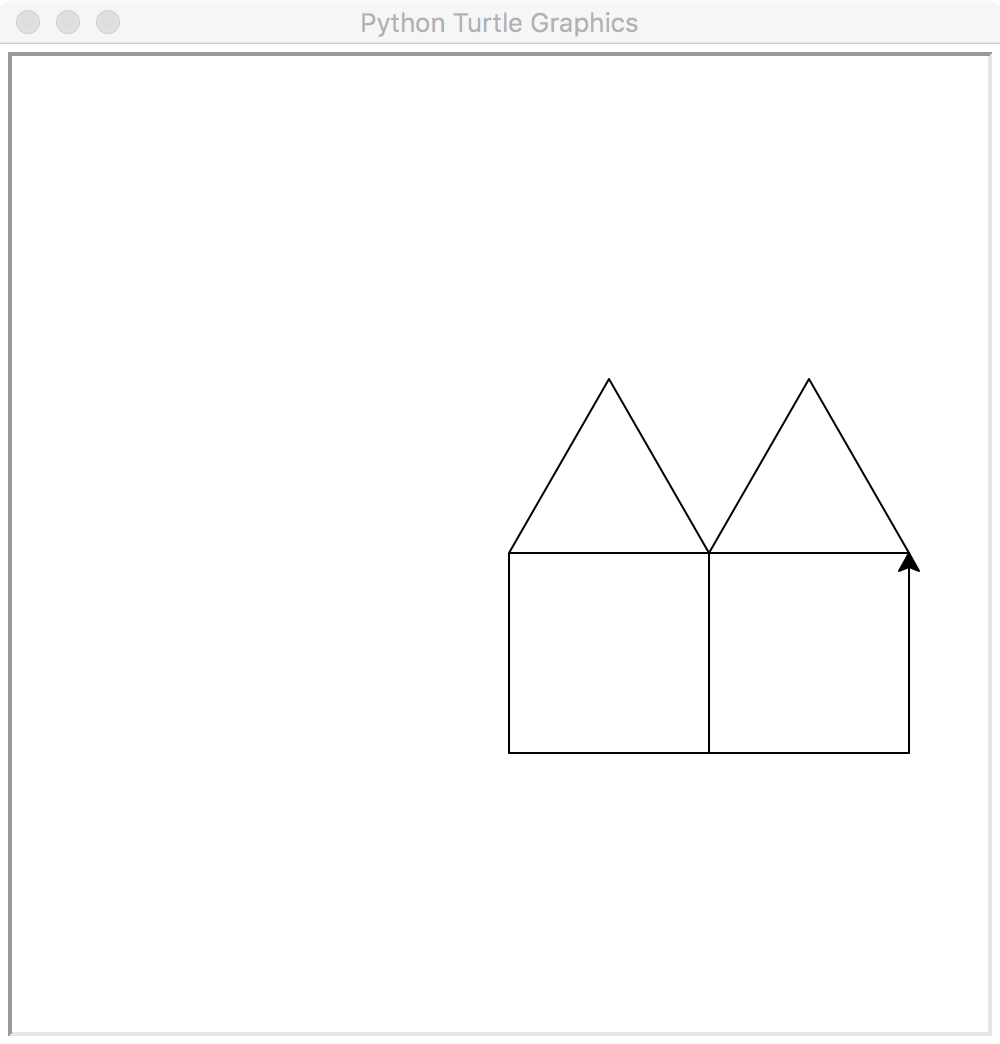# Answer:

 ```1 2 3``` ```house() tom.rt(90) house() ```

# Parameterizing the function

Wouldn't it be nice to draw houses of a different size, using the same function?

 ``` 1 2 3 4 5 6 7 8 9 10 11 12 13 14 15 16``` ```def house(size): tom.fd(size) tom.lt(120) tom.fd(size) tom.lt(120) tom.fd(size) tom.lt(30) tom.fd(size) tom.lt(90) tom.fd(size) tom.lt(90) tom.fd(size) house(50) tom.rt(90) house(50) ```

# Smaller townhouse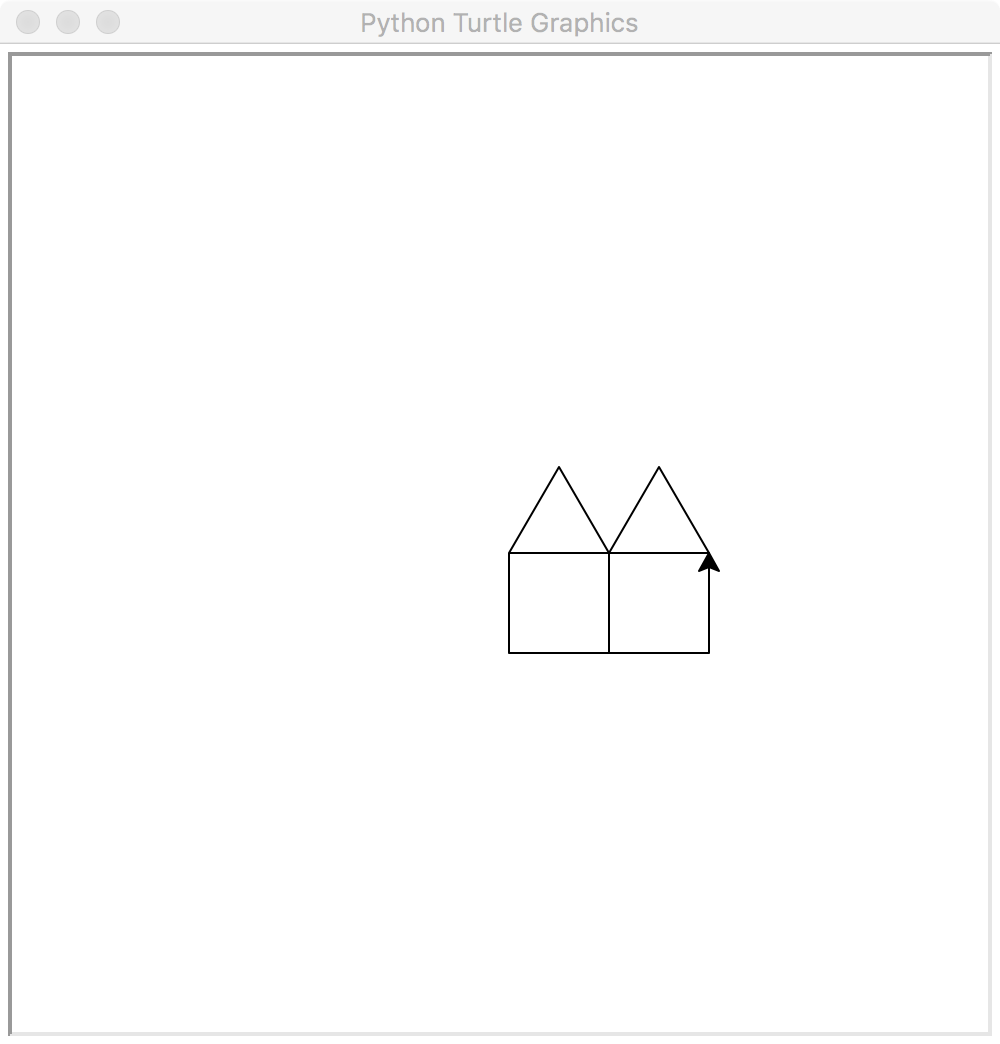# Exercise: Adding color

Hint: use `tom.fillcolor()` to set only the fill color.